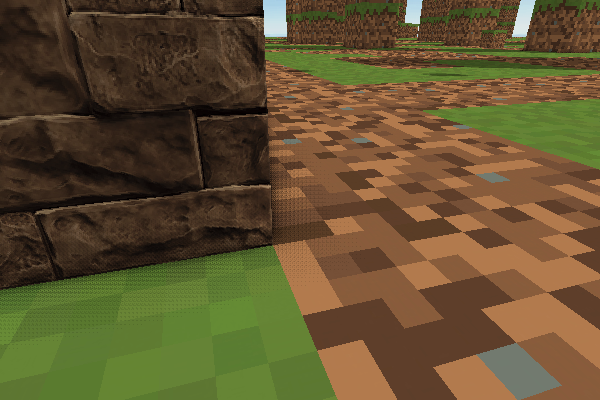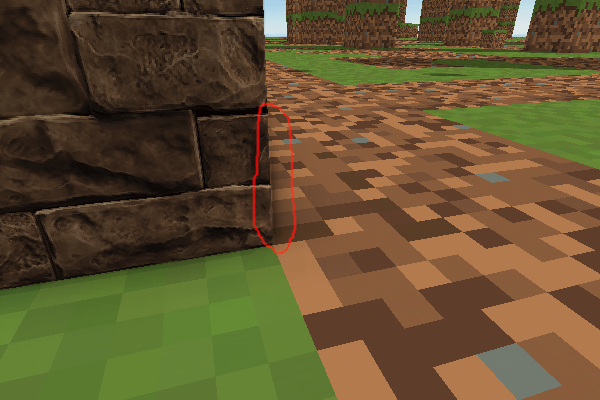# SSAO: Bilateral filter after AO path

Hello, there!

I try to implement SSAO.

There is a result:

AO path:blur path:As you can see after blur the image has light area that touches the wall at right.
Some research lets me know I should use ‘bilateral filter’ instead ‘simple blur’ to avoid such unrealistic fragments.

So I have found example: https://github.com/nvpro-samples/gl_ssao/blob/master/bilateralblur.frag.glsl

There are several questions related it:

``````...
layout(binding=0) uniform sampler2D texSource;
...``````

What is texSource?

``````...
layout(location=1) uniform vec2  g_InvResolutionDirection; // either set x to 1/width or y to 1/height
...
...

for (float r = 1; r <= KERNEL_RADIUS; ++r)
{
vec2 uv = texCoord + g_InvResolutionDirection * r;
c_total += BlurFunction(uv, r, center_c, center_d, w_total);
}

for (float r = 1; r <= KERNEL_RADIUS; ++r)
{
vec2 uv = texCoord - g_InvResolutionDirection * r;
c_total += BlurFunction(uv, r, center_c, center_d, w_total);
}
...``````

Why the algorithm goes diagonally?

Thanks for any help!